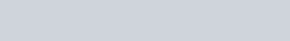# Logarithmic Series Distribution definition, formula, properties and applications

The logarithmic series distribution is a discrete probability distribution obtained from the Maclaurin series expansion. Sometimes it is known as log-series distribution. It has a long right tail with one parameter, which ranges from 0 to 1. The distribution is used to model a wide range of events, including data on animal diversity and the frequency of insurance claims.

## Logarithmic Series Distribution

A discrete random variable X is said to have logarithmic series distribution if its probability density function is defined as,where q is the parameter which lies between zero to one.

The name is justified as the probabilities for the various values of  x are the terms of the power-series expansion of ln(1-q). The distribution is obtained by truncating negative binomial distribution at x=0 and limiting r tends to zero.

### Properties

If the parameter of the Logarithmic series distribution is p, then the properties are-
• The mean of the Logarithmic Series Distribution is.
• The variance of the Logarithmic Series Distribution is.
• The mode of the Logarithmic Series Distribution is 1.

### Applications of Logarithmic Series Distribution

The logarithmic series distribution is used in different fields such as
• Biological science.
• Population growth.
• Economics.

### History of Logarithmic Series Distribution

The Logarithmic Series Distribution was first used systematically in a paper published in 1943 by Fisher, Corbet and Williams. It was, on the occasion, applied to the results of sampling butterflies and also to data obtained in connection with the collection of moths by means of a light trap.

Learn Data Science